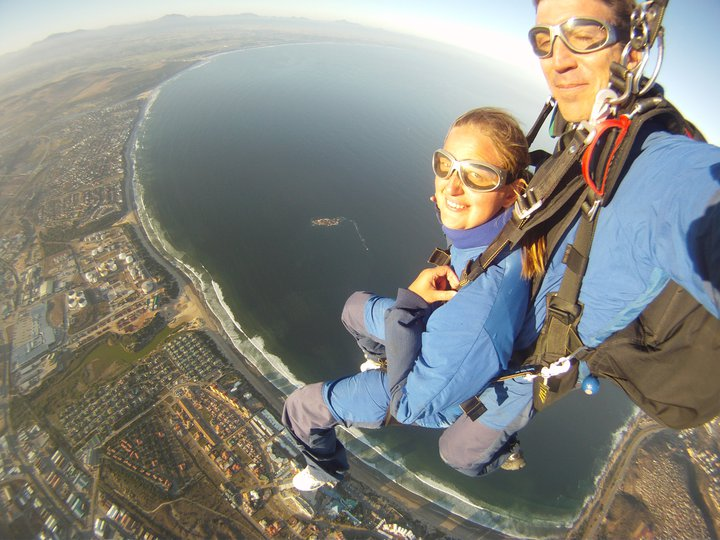Site Overlay#### Best Travels Around The World!

##### Africa

#06 = Gabon (2010)

#07 = Guinea (2010)

#08 = Equatorial Guinea (2010) / (2017) / (2018)

#14 = South Africa (2011) (2013)

#17 = Kenya (2011)

#18 = Tanzania; Island: Zanzibar (2011) / (2012)

#19 = Mozambique (2012)

#37 = Angola* (2012) / (2013)

#38 = Democratic Republic of Congo (2012)

#39 = Ethiopia (2012)

#57 = Namibia (2013)

#58 = Comoros (2014)

#60 = Senegal (2014)

#61 = Ghana (2017)

#62 = Morocco (2017)

#63 = Cameroon (2017)

#64 = Nigeria (2018)

#65 = Mauritania (2018)

##### Asia

#37 = United Arab Emirates (2012)

#72 = South Korea (2018)

#73 = Myanmar (2018) (2019)

#74 = China (2018) *Hong Kong

#75 = The Philippines (2018)

#76 = Indonesia (2018) (2019)

#77 = Timor-Leste (2018)

#78 = Brunei (2019)

#79 = Malaysia (2019)

#80 = Singapore (2019)

##### North America

#01 = United States of America (1983 – present)   ~48/50 US states visited

#02 = Canada (2000) / (2011)

#04 = The Bahamas; Islands: San Salvador, Nassau, Spanish Wells, Harbour Island, Eleuthera Island (2005) / (2011)

#05 = Mexico (2008) / (2011) / (2012)

#11 =Trinidad & Tobago (2010) / (2011)

U.S. Territory = Puerto Rico (2012)

French Territory = Martinique (2012)

Dutch Territory = Aruba (2013)

Central America:

#51 = Belize (2013)

#52 = Guatemala (2013)

#54 = Honduras (2013)

#55 = Nicaragua (2013)

#56 = Costa Rica (2013)

##### Europe

#03 = Scotland (2005) / (2014)

#09 = France (2010) / (2011) / (2012) / (2013)

#10 = Netherlands (2010) / (2013)

#12 = England (2010) / (2011)/ (2012)/ (2013) / (2014)

#13 = Wales (2011)

#15 = Northern Ireland (2011)

#16 = Republic of Ireland (2011)

#20 = Romania (2011)

#21 = Bulgaria (2011)

#22 = Serbia (2011)/ (2013)

#23 = Hungary (2011)

#24 = Croatia (2011)

#25 = Slovenia (2011)

#26 = Austria (2011)/ (2012)

#27 = Italy (2011) / (2013)

#28 = Monaco (2011)

#29 = Poland (2011)

#30 = Czech Republic (2011)

#31 = Switzerland (2011) / (2012)

#32 = Liechtenstein (2012)

#33 = Germany (2012)

#34 = Luxembourg (2012)

#35 = Belgium (2012)

#36 = Spain (2012)

#40 = Greece *Islands: Santorini Island, Mykonos, & Crete (2012) / (2013)

#41 = Russia (2012)

#43 = Bosnia & Herzegovina (2013)

#44 = Kosovo (2013)

#45 = Former Yugoslavia Republic of Macedonia (2013)

#46 = Albania (2013)

#47 = Montenegro (2013)

#48 = Turkey (2013)/ (2018)

#66 = Portugal (2018)

#67 = Estonia (2018)

#68 = Denmark (2018)

#69 = Norway (2018)

#70 = Sweden (2018)

#71 = Finland (2018)

##### South America

#42 = French Guiana (2012)

#50 = Suriname (2013)

#59 = Colombia (2014) / (2015) / (2016)

• Territories:

U.S. Territory = Puerto Rico (2012)

French Territory = Martinique (2012)

Dutch Territory = Aruba (2013)